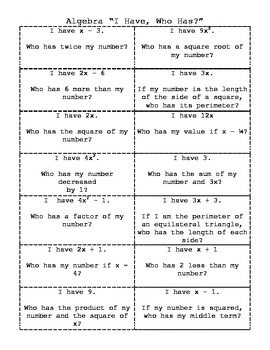Modeling 1 and 2 Step Equations - Entire Unit *Test Included*6th - 8th
Subjects
Standards
Resource Type
Formats Included
• Zip
Pages
49 pages

Description

This download includes everything you need to teach 1 and 2 step equations. Good resources can be hard and time consuming to find. It includes 16 resources bundled together. It is well aligned to the TEKS.

-Test and Review
-I Have You Have Cards
-Centers
-Partner Work
-Modeling With Algebra Tiles
-Worksheets
-Engaging Homework Worksheet Where Students Get to Draw

Total Pages
49 pages
N/A
Teaching Duration
2 Weeks
Report this Resource to TpT
Reported resources will be reviewed by our team. Report this resource to let us know if this resource violates TpT’s content guidelines.

Standards

to see state-specific standards (only available in the US).
Solve word problems leading to equations of the form 𝘱𝘹 + 𝘲 = 𝘳 and 𝘱(𝘹 + 𝘲) = 𝘳, where 𝘱, 𝘲, and 𝘳 are specific rational numbers. Solve equations of these forms fluently. Compare an algebraic solution to an arithmetic solution, identifying the sequence of the operations used in each approach. For example, the perimeter of a rectangle is 54 cm. Its length is 6 cm. What is its width?
Use variables to represent quantities in a real-world or mathematical problem, and construct simple equations and inequalities to solve problems by reasoning about the quantities.
Apply properties of operations as strategies to add, subtract, factor, and expand linear expressions with rational coefficients.